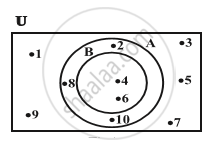# Venn Diagrams

#### notes

Most of the relationships between sets can be represented by means of diagrams which are known as Venn diagrams.  These diagrams consist of rectangles and  closed curves usually circles. The universal set is represented usually by a rectangle and its subsets by circles. In Venn diagrams, the elements of the sets are written in their respective circles
Example- In Fig, U = {1,2,3, ..., 10} is the universal set of which A = {2,4,6,8,10} and B = {4, 6} are subsets, and also B ⊂ A.If you would like to contribute notes or other learning material, please submit them using the button below.

### Shaalaa.com

Venn Diagrams - An Introduction [00:06:05]
S
0%